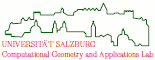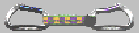# Generation of Random Polygonal Objects

Besides being a topic of interest of its own, the generation of random polygons has two main areas of application: a) testing the correctness and b) evaluating the CPU-time consumption of algorithms that operate on polygons. For testing the correctness of an algorithm, the goal is to generate a diverse set of input data such that all branches of the algorithm will be executed with a high probability. The same motivation applies to the practical testing of an algorithm's CPU-consumption. Ideally, one would like to test the performance of an algorithm on data of practical relevance. However, it often is next to impossible to obtain a sufficiently large number of practically relevant inputs. Then the second-best choice is to run an algorithm for a reasonably large number of random inputs.

This need for polygonal test data motivated Thomas Auer and me to study the generation of random polygons on a given set of vertices. Since no polynomial-time solution is known for the uniformly random generation of simple polygons, we have focused on heuristics that offer a good time complexity and still generate a rich variety of different polygons. Our algorithms were implemented by Thomas Auer and became part of RPG, our Random Polygon Generator. RPG currently features heuristics (and algorithms) for the generation of x-monotone, star-shaped, and general simple polygons. In addition, polygonal approximations of "random" free-form curves can be generated via a smoothing option.

More recently, Martin Heimlich ported RPG's GUI to GTK and added a neat heuristic for generating a "random" multiply-connected polygonal area for a given set of vertices. Roughly, we start with generating a random polygon on the set of vertices and then cut-off some of the pockets of the polygon by inserting parallel bridge edges, thus generating polygonal holes within a polygonal outer boundary. Furthermore, Michael Gschwandtner added code for geometric hashing in order to speed up the search for polygon edges that intersect pairwise. This new code provided a substantial improvement of the practical efficiency of those heuristics for the generation of random polygons that first generate an arbitrary polygon and then look for intersections, such as "2-Opt Moves".

RPG is available upon request. Please contact me, and let me know that you would like to get it.

Related publications:

T. Auer, M. Held (1996):
``Heuristics for the Generation of Random Polygons''.
Proc. 8th Canad. Conf. Computat. Geometry, Carleton University Press, F. Fiala, E. Kranakis, J.-R. Sack (eds.), pp. 38-44; Ottawa, Canada, Aug 12-15, 1996.

The following images show random polygons which were generated by means of RPG. (Click on an image icon in order to see the full-size image. The full-size images have 800x900 pixels.)This image shows a random star-shaped polygon with 50 vertices.This image shows a (truly) random x-monotone polygon with the same set of 50 vertices. (This polygon was constructed according to the algorithm of Chong Zhu, Gopalakrishnan Sundaram, Jack Snoeyink, and Joe Mitchell.)This image shows a "random" simple polygon with the same set of 50 vertices. The polygon was computed using RPG's "2-Opt Moves" heuristic.This image shows a "random" multiply-connected polygon with the same set of 50 vertices. (Holes are shown in cyan.)This image shows the result of "smoothing" the previous simply-connected polygon three times. (This is a fairly smooth polygon on 400 vertices.)This image shows the result of "smoothing" the previous multiply-connected polygon two times.This image shows a "random" simple polygon on a set of 200 clustered vertices. The polygon was computed using RPG's "2-Opt Moves" heuristic.This image shows the result of "smoothing" the previous polygon four times.This image shows a "random" simple polygon on a set of 10000 clustered vertices. The polygon was computed using RPG's "2-Opt Moves" heuristic.This image shows a zoomed view of the previous polygon.This image shows a zoomed view of a multiply-connected polygon on the same set of 10000 clustered vertices. (Holes are shown in cyan.)file last modified: Thursday, 27-Feb-2020 07:29:07 CETCopyright © 2020 Martin Held. All rights reserved.